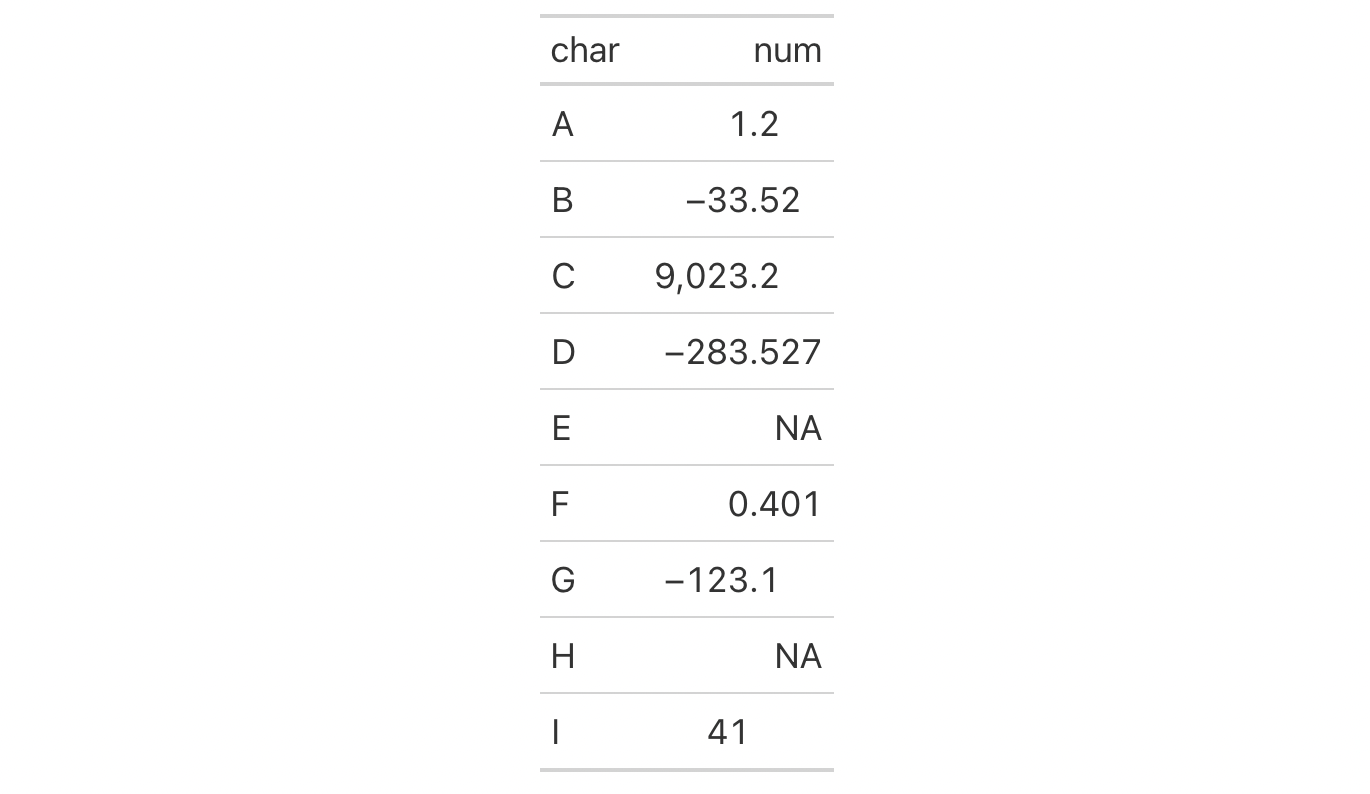For numeric columns that contain values with decimal portions, it is sometimes useful to have them lined up along the decimal mark for easier readability. We can do this with cols_align_decimal() and provide any number of columns (the function will skip over columns that don't require this type of alignment).

## Usage

cols_align_decimal(data, columns = everything(), dec_mark = ".", locale = NULL)

## Arguments

data

The gt table data object

obj:<gt_tbl> // required

This is the gt table object that is commonly created through use of the gt() function.

columns

Columns to target

<column-targeting expression> // default: everything()

The columns for which decimal alignment should be applied. Can either be a series of column names provided in c(), a vector of column indices, or a select helper function. Examples of select helper functions include starts_with(), ends_with(), contains(), matches(), one_of(), num_range(), and everything(). By default this is set to everything() which means that decimal alignment will be attempted on all columns.

dec_mark

Decimal mark

scalar<character> // default: "."

The character used as a decimal mark in the numeric values to be aligned. If a locale value was used when formatting the numeric values then locale is better to use and it will override any value here in dec_mark.

locale

Locale identifier

scalar<character> // default: NULL (optional)

An optional locale identifier that can be used to obtain the type of decimal mark used in the numeric values to be aligned (according to the locale's formatting rules). Examples include "en" for English (United States) and "fr" for French (France). We can use the info_locales() function as a useful reference for all of the locales that are supported. A locale ID can be also set in the initial gt() function call (where it would be used automatically by any function with a locale argument) but a locale value provided here will override that global locale.

## Value

An object of class gt_tbl.

## Examples

Let's put together a two-column table to create a gt table. The first column char just contains letters whereas the second column, num, has a collection of numbers and NA values. We could format the numbers with fmt_number() and elect to drop the trailing zeros past the decimal mark with drop_trailing_zeros = TRUE. This can leave formatted numbers that are hard to scan through because the decimal mark isn't fixed horizontally. We could remedy this and align the numbers by the decimal mark with cols_align_decimal().

dplyr::tibble(
char = LETTERS[1:9],
num = c(1.2, -33.52, 9023.2, -283.527, NA, 0.401, -123.1, NA, 41)
) |>
gt() |>
fmt_number(
columns = num,
decimals = 3,
drop_trailing_zeros = TRUE
) |>
cols_align_decimal()5-2

## Function Introduced

v0.8.0 (November 16, 2022)

Other column modification functions: cols_add(), cols_align(), cols_hide(), cols_label_with(), cols_label(), cols_merge_n_pct(), cols_merge_range(), cols_merge_uncert(), cols_merge(), cols_move_to_end(), cols_move_to_start(), cols_move(), cols_nanoplot(), cols_unhide(), cols_units(), cols_width()